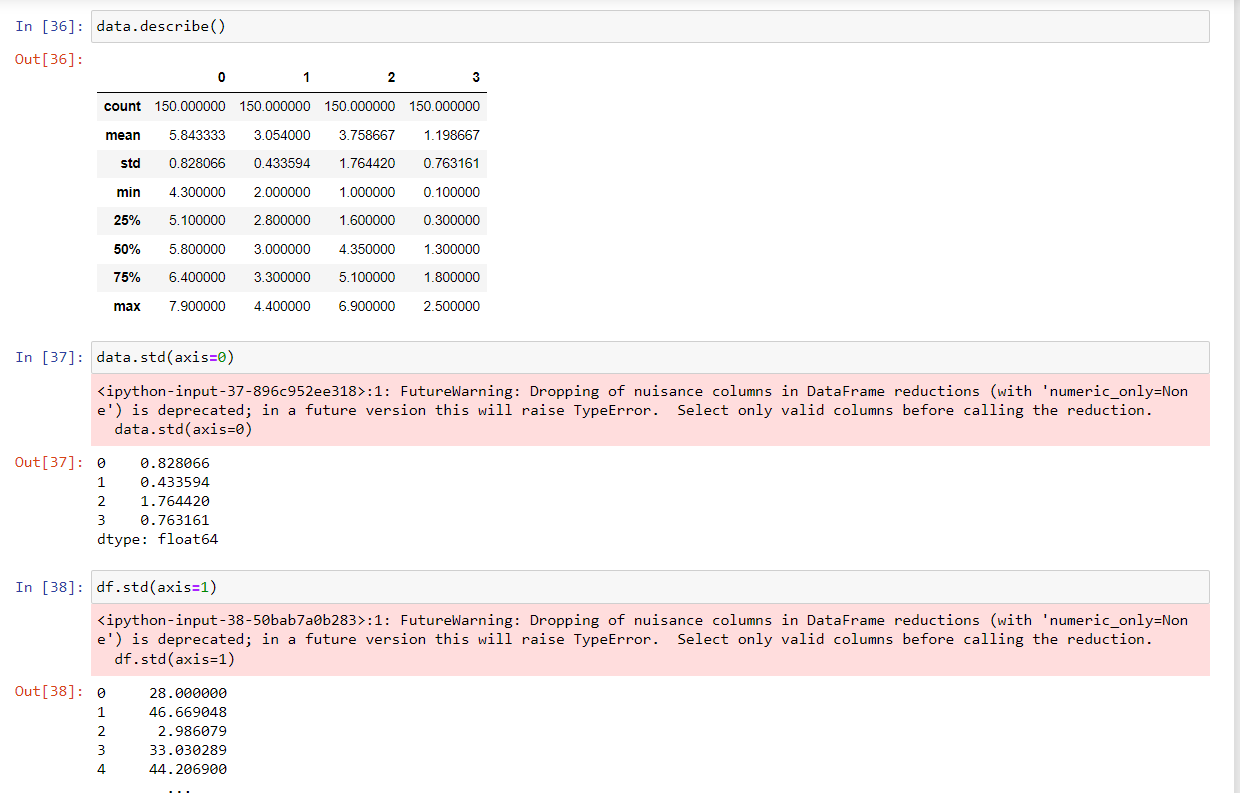# How to Calculate Summary Statistics for a Pandas DataFrameby ganeshUpdated: Jan 24, 2023Solution Kit

Summary statistics are statistical measures that summarize or describe a set of observations. In the context of a pandas DataFrame, summary statistics are statistical measures that summarize the data in the DataFrame.

A pandas DataFrame's describe() function allows you to generate a number of summary statistics for the data. This function provides a new DataFrame with a row for each numerical column and a column for each statistic, along with the statistical summary of the data.

• describe(): A number of summary statistics, including as the count, mean, standard deviation, minimum, maximum, and quartiles of the data, are computed by default using the describe() method. You may select which columns to include in the summary using the include option and which columns to omit using the exclude parameter.

Other techniques, including mean(), median(), min(), max(), and std(), can be used to obtain certain summary statistics for the data.

Here is how to calculate summary statistics for a Pandas DataFrame;Fig 1: Preview of the output that you will get on running this code from your Jupyter notebook

### Code

In this solution, we use the describe function of the Pandas library

1. Copy the code using the "Copy" button above, and paste it in a cell of Jupyter notebook.
2. Run the cell to read online data and create a Pandas dataframe.

I hope you found this useful. I have added the link to dependent libraries, version information in the following sections.

I found this code snippet by searching for "pandas summary statistics" in kandi. You can try any such use case!

### Dependent Libraries

pandasby pandas-dev

Python38499Version:v2.0.2Flexible and powerful data analysis / manipulation library for Python, providing labeled data structures similar to R data.frame objects, statistical functions, and much more

Support
Quality
Security
Reuse

pandasby pandas-dev

Python38499Version:v2.0.2License: Permissive (BSD-3-Clause)

Flexible and powerful data analysis / manipulation library for Python, providing labeled data structures similar to R data.frame objects, statistical functions, and much more
Support
Quality
Security
Reuse

If you do not have Pandas that is required to run this code, you can install it by clicking on the above link and following the installation instruction from either Github or Pypi links through the Pandas page in kandi.

You can search for any dependent library on kandi like Pandas.

### Environment Tested

I tested this solution in the following versions. Be mindful of changes when working with other versions.

1. The solution is created in Python3.7.
2. The solution is tested on Pandas 1.3.1 version.

Using this solution, we are able to create summary statistics of a Dataframe using the Pandas library in Python with simple steps. This process also facilities an easy to use, hassle free method to create a hands-on working version of code which would help us read data in Pandas.

### Support

1. For any support on kandi solution kits, please use the chat
2. For further learning resources, visit the Open Weaver Community learning page.

See similar Kits and Libraries